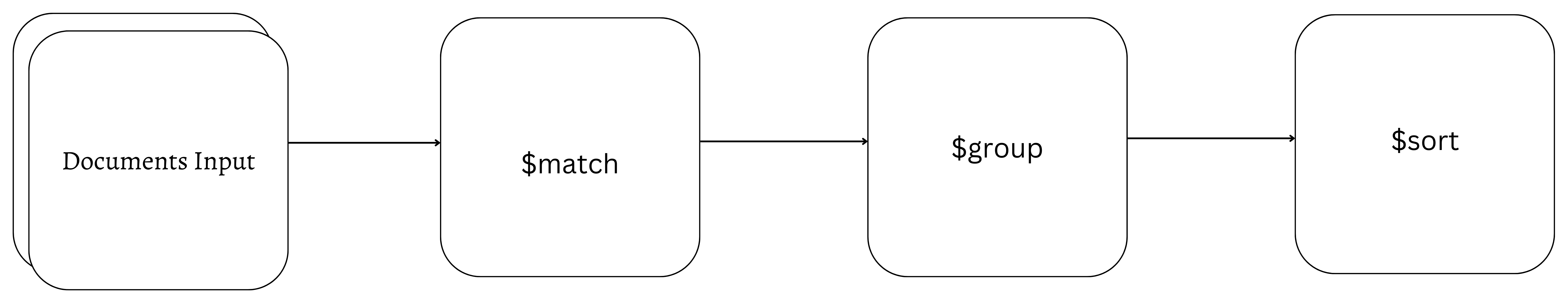# Aggregation pipeline and commands

Aggregation operations involve performing various operations on a large number of data records, such as data grouping, sorting, restructuring, or modifying. These operations pass through one or more stages, which make up a pipeline.Each stage acts upon the returned documents of the previous stage, starting with the input documents. As shown above, the documents pass through the pipeline with the result of the previous stage acting as input for the next stage, going from `\$match` => `\$group` => `\$sort` stage.

For example, insert the following documents in a `sales` collection:

``db.sales.insertMany([  { _id: 1, category: 'Electronics', price: 1000 },  { _id: 2, category: 'Electronics', price: 800 },  { _id: 3, category: 'Clothing', price: 30 },  { _id: 4, category: 'Clothing', price: 50 },  { _id: 5, category: 'Home', price: 1500 },  { _id: 6, category: 'Home', price: 1200 },  { _id: 7, category: 'Books', price: 20 },  { _id: 8, category: 'Books', price: 40 }])``

A typical aggregation pipeline would look like this:

``db.sales.aggregate([  { \$match: { category: { \$ne: 'Electronics' } } },  {    \$group: {      _id: '\$category',      totalPrice: { \$sum: '\$price' },      productCount: { \$sum: 1 }    }  },  { \$sort: { totalPrice: -1 } }])``

In the pipeline, the complex query is broken down into separate stages where the record goes through a series of transformations until it finally produces the desired result. First, the `\$match` stage filters out all documents where the `category` field is not `Electronics`. Then, the `\$group` stage groups the documents by their `category` and calculates the total price and product count for each of those category. Finally, the `\$sort` stage sorts the documents by the `totalPrice` field in descending order.

So the above aggregation pipeline operation would return the following result:

``[  { _id: 'Home', totalPrice: 2700, productCount: 2 },  { _id: 'Clothing', totalPrice: 80, productCount: 2 },  { _id: 'Books', totalPrice: 60, productCount: 2 }]``

This section of the documentation will focus on `aggregate` command, aggregation stages, and aggregation operators.

## `aggregate` command​

The aggregation command `aggregate` is a top-level command used for aggregating data across various pipeline stages.

The command is used for performing aggregation operations on a collection and lets you specify aggregation operations in a pipeline consisting of one or more stages and operators for transforming and analyzing data, such as grouping, filtering, sorting, projecting, and calculating aggregates.

``// Aggregation pipeline to perform aggregation operations on a collectiondb.collection.aggregate([  // Stage 1: Matching documents based on a specific field and value  { \$match: { field: value } },  // Stage 2: Grouping documents by the "category" field and calculating the sum of the "quantity" field  { \$group: { _id: '\$category', total: { \$sum: '\$quantity' } } }])``